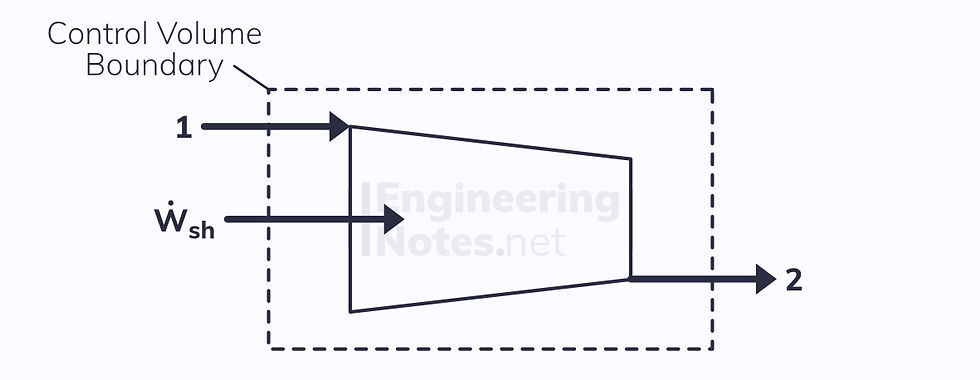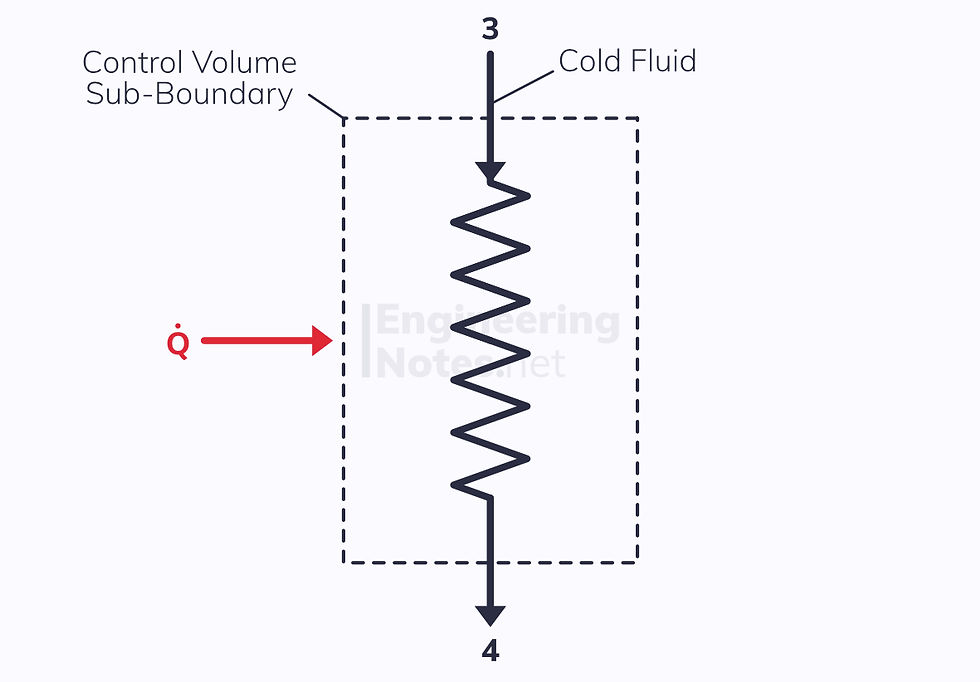top of page

#### Notes by Category University Engineering

Electronics*
Mathematics*
Mechanics & Stress Analysis*
Rate these notesNot a fanNot so goodGoodVery goodBrillRate these notes

In this notes sheet:

The basic form of the first law, ΔQ – ΔW = ΔE, only applies to closed systems - no mass can transfer across the system boundary, only energy in the form of heat and work.

In reality, perfectly closed systems are quite rare (take a turbine, for example: air flows in as well as heat, shaft work and hot air flow out), and as such a different model is required:The energy transfers across the control volume surface are:

• Shaft work

• Heat transfer

• Energy in the working fluid (kinetic, potential, and internal energies)

To simplify the process, we only look at the energy inputs and outputs: what goes on inside the control volume is irrelevant.

We call where the working fluid enters and exits ports.

• The control volume above has two ports: one inflow and one outflow port.

## The Steady Flow Energy Equation (SFEE)

In order to solve problems involving steady flow through a control volume, we use the Steady Flow Energy Equation (SFEE) instead of the simple first law equation:In a less mathematically intimidating form:

• Left hand side: rate of energy transfer – rate of shaft work

• Right hand side: sum of output mass energy flow rates – sum of input mass energy flow rates

Note: The dot above the letters means it is a rate of change with respect to time:Thankfully, kinetic and potential energies can often be ignored, simplifying the equation immensely. However, it is important you start with the above form when solving problems, else you will likely forget parts.

See the derivation of the SFEE here

### Mass Continuity Equation

We can use the SFEE in conjunction with the principle of conservation of mass. This means that the mass in the control volume must stay constant, so the total input mass flow rate = total output mass flow rate:Therefore, for a two-port control volume where potential energies are negligible, the SFEE reduces to:When kinetic energy too can be ignored, it becomes:The point is, the SFEE in its full form may look difficult, but it really isn’t. Just be neat and methodical in your workings, and always start from the full form and then see which terms you can set to zero.

## Applications of the SFEE

There are a number of very common examples where the SFEE is applied and simplified. These are worth knowing, as crop up in almost all problems involving steady flow.

This is also where some actual real-life engineering gets involved. Which is fun.

Each example has a standard block diagram symbol. These should be learned as they are used to describe multi-stage processes like the Rankine Cycle (see below).

### Partly Closed Valve (Throttling)This is the simplest form every term in the SFEE other than the enthalpies are negligible:

• Heat transfer is negligible when the valve is well insulated

• There is no shaft work

• Kinetic energy change is negligible

• Potential energy change is negligible

There are two ports, so the SFEE reduces to:From this you can see that throttling (the process that takes place in a partly-closed valve) is isenthalpic.

### NozzlesA simple nozzle can be modelled as a duct of reducing cross-sectional area, used to increase the kinetic energy of the working fluid at the expense of its enthalpy.

• There is no heat transfer to or from the working fluid

• There is no shaft work

• There is a massive change in kinetic energy

• Potential energy is negligible

Again, there are two ports, so the SFEE becomes:### BoilersHeat is transferred to the working fluid to the point of saturation, and the fluid evaporates.

Boilers are isobaric, meaning there is no work done.
• Kinetic energy is negligible

• Potential energy is negligible

There are two ports, so the SFEE reduces to:### Pumps & CompressorsPumps and compressors are exactly the same thing: the only difference is that a pump refers to a liquid and a compressor refers to a gas (as liquids are generally incompressible).

• There is typically no heat transfer – the process is adiabatic

• There is a pressure change, so there is shaft work

• Kinetic energy and potential energy can sometimes be ignored, but not always

When KE and PE are negligible, the SFEE reduces to:If KE and PE are not negligible (e.g. pumping water up a hill), the SFEE becomes:Where v is the specific volume. In this instance, specific work is given by vΔP, not PΔv.

### TurbinesThe opposite of a pump or compressor, the working fluid expands in a turbine to provide shaft work.

• Heat transfer is generally negligible

• There is a lot of output shaft work (h₁ > h₂, so W > 0)

• Kinetic energy is sometimes negligible, but often not

• Potential energy can usually be ignored

Two ports, so the SFEE becomes:### Heat ExchangerA heat exchanger (as its name suggests) transfers heat from one working fluid to another. This means that if we make the control volume the whole exchanger with four ports, there is no net heat transfer.

Obviously, there is also no shaft work or change in kinetic and potential energy. Therefore:Using mass continuity equations for ports 1 & 2 and 3 & 4, we can simplify the SFEE even further to:However, if we make the control volume just one of the two fluids with a positive or negative heat transfer from the other fluid, there are two ports and there is a heat transfer:Where the heat transfer comes from the hot stream: